Test: State Variable Analysis - 1

# Test: State Variable Analysis - 1 - Electrical Engineering (EE)

Test Description

## 10 Questions MCQ Test GATE Electrical Engineering (EE) 2024 Mock Test Series - Test: State Variable Analysis - 1

Test: State Variable Analysis - 1 for Electrical Engineering (EE) 2023 is part of GATE Electrical Engineering (EE) 2024 Mock Test Series preparation. The Test: State Variable Analysis - 1 questions and answers have been prepared according to the Electrical Engineering (EE) exam syllabus.The Test: State Variable Analysis - 1 MCQs are made for Electrical Engineering (EE) 2023 Exam. Find important definitions, questions, notes, meanings, examples, exercises, MCQs and online tests for Test: State Variable Analysis - 1 below.
Solutions of Test: State Variable Analysis - 1 questions in English are available as part of our GATE Electrical Engineering (EE) 2024 Mock Test Series for Electrical Engineering (EE) & Test: State Variable Analysis - 1 solutions in Hindi for GATE Electrical Engineering (EE) 2024 Mock Test Series course. Download more important topics, notes, lectures and mock test series for Electrical Engineering (EE) Exam by signing up for free. Attempt Test: State Variable Analysis - 1 | 10 questions in 30 minutes | Mock test for Electrical Engineering (EE) preparation | Free important questions MCQ to study GATE Electrical Engineering (EE) 2024 Mock Test Series for Electrical Engineering (EE) Exam | Download free PDF with solutions
 1 Crore+ students have signed up on EduRev. Have you?
Test: State Variable Analysis - 1 - Question 1

### Which one of the following information is necessary to formulate the problem of control systems optimization?

Test: State Variable Analysis - 1 - Question 2

### For arbitrary pole placement, the following combination is necessary.

Test: State Variable Analysis - 1 - Question 3

### For a linear time invariant system, an optimal controller can be designed if

Detailed Solution for Test: State Variable Analysis - 1 - Question 3

An optimal controller for an LTI system can be designed provided the system is both controllable and observable

Test: State Variable Analysis - 1 - Question 4

The eigen values of linear system are the location of

Detailed Solution for Test: State Variable Analysis - 1 - Question 4

Eigen value are given by | sI - A | = 0, which is the location of poles.

Test: State Variable Analysis - 1 - Question 5

If the system matrix of a linear time invariant continuous system is given by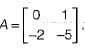Its characteristic equation will be given by

Detailed Solution for Test: State Variable Analysis - 1 - Question 5

Characteristic equation is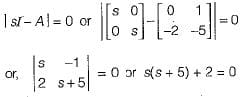or, s2 + 5s + 2 = 0

Test: State Variable Analysis - 1 - Question 6

​The state-variable description of a linear autonomous system is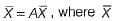is a two-dimensional state vector and A is a matrix given by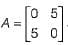The poles of the system are located at

Detailed Solution for Test: State Variable Analysis - 1 - Question 6

Poles of given system are given by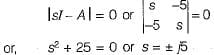Test: State Variable Analysis - 1 - Question 7

Assertion (A): The eigen values of a linear continuous-data time invariant system controls the stability of the system.
Reason (R): The roots of the characteristic equation are the same as the eigen values of system matrix A of the state equations.

Test: State Variable Analysis - 1 - Question 8

The vector matrix differential equation of a system is given by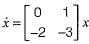The state transition matrix of the system is

Detailed Solution for Test: State Variable Analysis - 1 - Question 8

We have: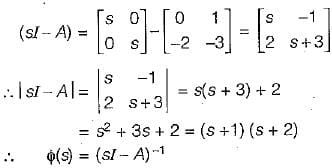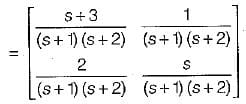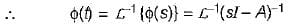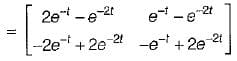Test: State Variable Analysis - 1 - Question 9

The system matrix of a continous time system is given by: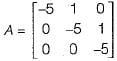​The characteristic equation is

Detailed Solution for Test: State Variable Analysis - 1 - Question 9

The characteristic equation | sI - A | = 0.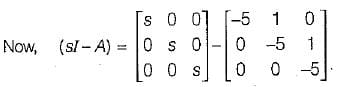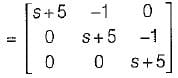Now, | sI - A | = 0
or, (s + 5) [(s + 5)2 + 0] + 1 (0 - 0) + 0 = 0
or, (s + 5)3 = 0 or s3 + 15s2 + 75s + 125 = 0

Test: State Variable Analysis - 1 - Question 10

A system is described by the following equations: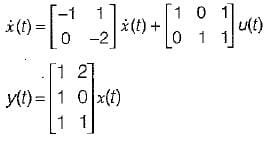The transfer function of the system is

Detailed Solution for Test: State Variable Analysis - 1 - Question 10

Transfer function is given by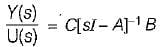Given,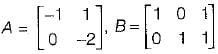and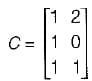Now,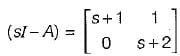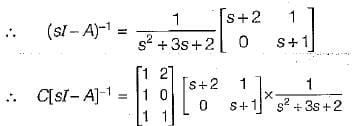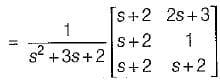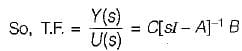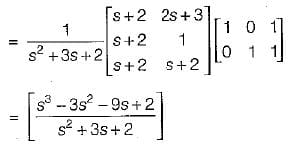## GATE Electrical Engineering (EE) 2024 Mock Test Series

23 docs|285 tests
Information about Test: State Variable Analysis - 1 Page
In this test you can find the Exam questions for Test: State Variable Analysis - 1 solved & explained in the simplest way possible. Besides giving Questions and answers for Test: State Variable Analysis - 1, EduRev gives you an ample number of Online tests for practice

## GATE Electrical Engineering (EE) 2024 Mock Test Series

23 docs|285 tests